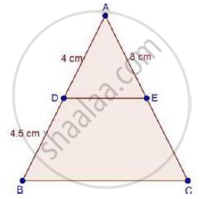Share

Books Shortlist
Your shortlist is empty

# Solution for In δAbc, D and E Are Points on the Sides Ab and Ac Respectively Such that De || Bc If Ad = 4 Cm, Db = 4.5 Cm and Ae = 8 Cm, Find Ac. - CBSE Class 10 - Mathematics

ConceptBasic Proportionality Theorem Or Thales Theorem

#### Question

In ΔABC, D and E are points on the sides AB and AC respectively such that DE || BC

If AD = 4 cm, DB = 4.5 cm and AE = 8 cm, find AC.

#### SolutionWe have,

DE || BC

Therefore, by basic proportionality theorem, we have,

"AD"/"DB"="AE"/"EC"

rArr4/4.5=8/"EC"

rArr"EC"=(8xx4.5)/4

⇒ EC = 9cm

Now, AC = AE + EC

= 8 + 9

= 17 cm

∴ AC = 17 cm

Is there an error in this question or solution?

#### Video TutorialsVIEW ALL 

Solution for question: In δAbc, D and E Are Points on the Sides Ab and Ac Respectively Such that De || Bc If Ad = 4 Cm, Db = 4.5 Cm and Ae = 8 Cm, Find Ac. concept: Basic Proportionality Theorem Or Thales Theorem. For the course CBSE
S